# Angle: The angles of elevation of the top of the tree was viewed by two boys as shown....

#### chijioke

##### Full Member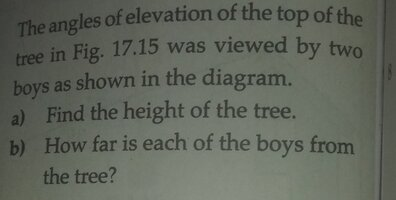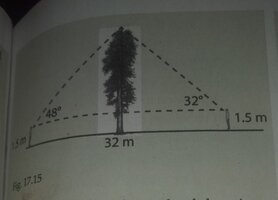This is what I did. I thought that the big triangle is bisected into two equal triangles thereby bisecting the distance 32m into 16m each.View attachment 35764
Having this in mind,
Let h be the upper part of the tree above the boys head.
From triangle ABC, $tan48=\frac{h}{16}$$h=16tan48=17.769...~m$Complete height of the tree becomes
$\therefore~h_c=17.769+1.5=19~m$I wanted to be sure that the two boys are observing the height as the same, so I calculated the height from triangle DEF. $tan32=\frac{h}{16}$ $h=16tan32=9.997~m$Complete height of the tree
$h_c=9.997+1.5=11~m$Ohoo ohoo!
Height is not the same from the two triangles. I must have gone wrong some where. Where could this error have come from?

Last edited:

#### blamocur

##### Senior Member
I thought that the big triangle is bisected into two equal triangles thereby bisecting the distance 32m into 16m each.
Why do you think the two triangles are equal ?? It is a good idea to double-check that the height is the same in both triangles -- this way you showed that you assumption about the triangles' equality is flawed.

•NotJeffM

#### Dr.Peterson

##### Elite Member
I thought that the big triangle is bisected into two equal triangles thereby bisecting the distance 32m into 16m each.
Now you know that you were wrong. (The fact that they asked you to find those two distances after finding the height should also have been a clue ... along with everyday experience that if you have to look up more at a building, you are closer to it).

So try using three variables: h for the tree's height, and x and y for the two horizontal distances. Write an equation that says x+y=32, rather than assume x=y=16.

And come away with lesson number one: Never Assume Anything. Always have a reason.

•NotJeffM

#### NotJeffM

##### Junior Member
The two triangles cannot be copies of one another: they are not even similar triangles because they explicitly contain different angles.

It is, as both preceding responses acknowledged, great that you checked your work in the only way possible.

#### The Highlander

##### Full Member
View attachment 35749View attachment 35753 This is what I did. I thought that the big triangle is bisected into two equal triangles thereby bisecting the distance 32m into 16m each.View attachment 35764
Having this in mind,
Let h be the upper part of the tree above the boys head.
From triangle ABC, $tan48=\frac{h}{16}$$h=16tan48=17.769...~m$Complete height of the tree becomes
$\therefore~h_c=17.769+1.5=19~m$I wanted to be sure that the two boys are observing the height as the same, so I calculated the height from triangle DEF. $tan32=\frac{h}{16}$ $h=16tan32=9.997~m$Complete height of the tree
$h_c=9.997+1.5=11~m$Ohoo ohoo!
Height is not the same from the two triangles. I must have gone wrong some where. Where could this error have come from?
Once again you are just ignoring the advice given to you!

What was the last thing I told you?

That you should start all these problems by drawing a sketch!

Again, I have done it for you...

and now that you have a sketch of the problem you can (surely?) see what ∠ACB is and it is then a simple matter to calculate the length of AC (or BC) and thence h. (Using a certain Law with which I know you are familiar and Basic Right-Angled Triangle Trigonometry!)

The height of the tree is, of course,
h+1.5m and once you have gone thus far you should have no difficulty in finding the lengths of AT & BT.

Last edited:

#### The Highlander

##### Full Member
And, please stop saying things are equal when they are not equal at all!

For example...$h=16tan32\not=9.997~m$$h=16tan32\approx9.998~m$
(You didn't even round it correctly!)
and, you should have written...

$$\displaystyle h_c\approx9.998+1.5\approx\underline{\underline{11~m}}~$$ or $$\displaystyle ~h_c\approx9.998+1.5=\underline{\underline{11~m}}~$$(to 2 s.f.)

Last edited:

#### chijioke

##### Full Member
And, please stop saying things are equal when they are not equal at all!

For example...$h=16tan32\not=9.997~m$$h=16tan32\approx9.998~m$
(You didn't even round it correctly!)
and, you should have written...

$$\displaystyle h_c\approx9.998+1.5\approx\underline{\underline{11~m}}~$$ or $$\displaystyle ~h_c\approx9.998+1.5=\underline{\underline{11~m}}~$$(to 2 s.f.)
Noted
Once again you are just ignoring the advice given to you!

What was the last thing I told you?

That you should start all these problems by drawing a sketch!
Yes I already did one but could not successfully upload it at that time.
Again, I have done it for you...

Ok thank you for the diagram. But I am seeing something in the diagram. It looks as if |TB| = 32m; while in the main diagram, |AB| = 32m. So what actually happen?

and now that you have a sketch of the problem you can (surely?) see what ∠ACB is and it is then a simple matter to calculate the length of AC (or BC) and thence h. (Using a certain Law with which I know you are familiar and Basic Right-Angled Triangle Trigonometry!)
Yes, That I shall do and I wiill show it here.
The height of the tree is, of course, h+1.5m and once you have gone thus far you should have no difficulty in finding the lengths of AT & BT
Yes. Very true.
Just incase you are so much interested in seeing the earlier sketches
or interpretation I tried making before I could not successfully upload it. Here is it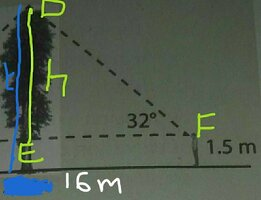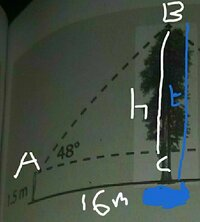Though you had told me earliar that |AC| and |EF| are not 16m ( which agree immedieately as I could not get the same value for height from both triangle)
The two triangles cannot be copies of one another: they are not even similar triangles because they explicitly contain different angles.

It is, as both preceding responses acknowledged, great that you checked your work in the only way possible.
Yes.
Why do you think the two triangles are equal ??
The tree in the middle was making me to think so.
It is a good idea to double-check that the height is the same in both triangles -- this way you showed that you assumption about the triangles' equality is flawed.
Yes that is why I finally came for help.
Now you know that you were wrong. (The fact that they asked you to find those two distances after finding the height should also have been a clue ... along with everyday experience that if you have to look up more at a building, you are closer to it).
Yes. Very true.
So try using three variables: h for the tree's height, and x and y for the two horizontal distances. Write an equation that says x+y=32, rather than assume x=y=16.
That I will try to do and see where it will lead me to and I shall show it once I am true.
And come away with lesson number one: Never Assume Anything. Always have a reason.
Yes. Very true.

#### blamocur

##### Senior Member
Ok thank you for the diagram. But I am seeing something in the diagram. It looks as if |TB| = 32m; while in the main diagram, |AB| = 32m. So what actually happen?
It might look slightly misleading, but having I am sure @The Highlander meant the AB, not TB. Note that the sides of the large triangle are labeled [imath]a,b,c[/imath], which make me things that "c(=32m)" refers to the whole side AB.

•chijioke

#### chijioke

##### Full Member
It might look slightly misleading, but having I am sure @The Highlander meant the AB, not TB. Note that the sides of the large triangle are labeled [imath]a,b,c[/imath], which make me things that "c(=32m)" refers to the whole side AB.
Ok. Thank you. I will give solving a try.

#### chijioke

##### Full Member
Same mistake that was made in post #5 is repeated again. I said that |AB| = 32m. So $|TB|\ne32m-x$ that is$|TB|\ne 32-|AT|$ rather $|AB|=|AT|+|TB|=32m$ Please take a look at the diagram in post #1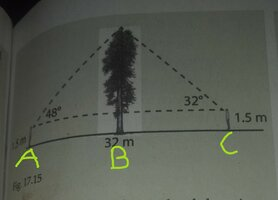Where labelled |AT| as |AB| and |AB| as |AC|. So you can see from it that |AC|=|AB|+|BC|=32m. Can you see it?

#### khansaheb

##### Full Member
Same mistake that was made in post #5 is repeated again. I said that |AB| = 32m.
No - the side opposite to vertex 'C' is called 'c' .

Where labelled |AT| as |AB| and |AB| as |AC|. So you can see from it that |AC|=|AB|+|BC|=32m.
That is what is claimed in the diagram of Highlander (which was repurposed in and the calculations in #10. According to the diagram in #10,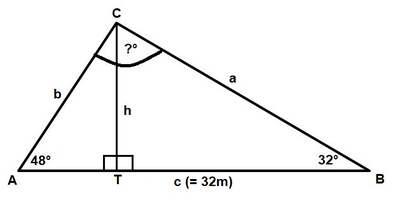AB (=c) = 32

AB = AT(=x) + TB = x + (32-x)

Please review #10 with a pencil paper and redo it, step-by-step.

#### The Highlander

##### Full Member
Noted

Yes I already did one but could not successfully upload it at that time.
No! Looking at what you post below, these are not (your) sketches!
Attempting to add dimensions onto the diagram in the original question is not the same as drawing your own sketch!
The diagram in the question is clearly inaccurate (quite possibly deliberately so, to encourage unwary students to make the same mistakes as you did?) and that is why it is important that you make your own sketch of the problem.
A sketch does not need to be geometrically accurate (mine isn't; those angles are not 32° or 48°!) though it does no harm to make it as close as possible; the use of a protractor &straight edge would allow the construction of a more accurate sketch than mine but what is important is that the sketch is correctly labelled and all the correct dimensions are added in the correct places.
If you had just started by drawing a triangle similar to the (isosceles?) one in the question, once you added in the angles it would become obvious (I would hope) that it wasn't the correct geometry and you would then be 'prompted' to redraw the triangle to be more like my sketch.
However, even if you didn't re-draw the triangle (as scalene rather than isosceles) but did correctly label it (as in my sketch) that would still enable you to correctly answer the question by just ignoring the incorrect geometry and using the information provided (in the sketch) in the correct way!

Ok thank you for the diagram. But I am seeing something in the diagram. It looks as if |TB| = 32m; while in the main diagram, |AB| = 32m. So what actually happen?
There is a (to the best of my knowledge &belief) universally accepted convention for labelling triangles; are you not familiar with this? (If not, then you need to read this!) To summarize: the vertices are labelled with capital letters (eg: A, B & C) and the side opposite each vertex is labelled with the corresponding lower-case letter (ie: a, b & c); in a right-angled triangle the right-angle usually takes the third (in alphabetical order) letter (so that a2 + b2 = c2).
That is why (in my sketch) c represents the line AB, not any segment of it, and also why I added "= 32m" in brackets thus making it absolutely clear what length it was so there should have been no confusion whatsoever as to what c was (for anyone paying any attention)!
The point T was added simply to facilitate the naming of the two distances the boys were from the tree (as AT & BT). I suppose I might have put that label inside the triangle but the little squares (identifying the right angles) were already there and I very much doubt that anyone else looking at that diagram would have misinterpreted it the way you appear to have!
Just incase you are so much interested in seeing the earlier sketches
or interpretation I tried making before I could not successfully upload it. Here is it
View attachment 35769
View attachment 35770
Those are not sketches. As explained earlier, you should not be just adding dimensions to a 'provided' diagram but drawing your own sketch of a situation. That way you are thinking about the situation you are faced with and (especially in this case) are likely to realise the problem with the original diagram in the question!

draw your own sketches in future (you can just use mine in this case).

NB: They don't have to be geometrically accurate (although it's better if they at least approximate the correct geometry, even if that involves
re-drawing a figure once or twice) and they can be hand drawn (in pencil?) rather than created digitally if that's easier for you. The most important thing about any sketch is that is correctly labelled and shows all the available information (lengths, angle measures, etc.) in the correct places; that will make it so much simpler to think about how to approach the solution of a problem (and avoid simple 'traps' that the question setter(s) may be encouraging you to fall into).

#### The Highlander

##### Full Member
h = 32/[cot(32) + cot(48)]..............................................How would you check this answer??
He could "check" it by calculating "h" using the much simpler approach outlined at Post #5 (q.v.)!

Did you not read all the way through that post (before you nicked my diagram?).

Are you deliberately trying to confuse the OP (even further)?

All he has to do is state the measure of
∠ACB (knowing that angles in a triangle add to 180°) which then enables him to calculate the length of a (and/or b) via the Law of Sines (which he has used in previous posts) and then it's a simple matter to find the length "h" because...$Sine~32° =\frac{h}{a}\;\;\;\text{and/or}\;\;\; Sine~48°=\frac{h}{b}$Having (thus) calculated "h" it is then another simple matter to calculate the the lengths of AT & BT via the Tangent ratios of the given angles.

There is absolutely no need to introduce any complicated algebraic manipulation or, indeed, any inverse trigonometric ratios, which might well tax the OP's understanding and capabilities, thank you.

#### The Highlander

##### Full Member
Same mistake that was made in post #5 is repeated again.
There was NO "mistake... made in post #5"!!!!
The only mistake made was your misreading of a perfectly clear diagram supplied to aid your understanding of the problem and clear up your misconceptions. You really need to take on board the advice you are being offered instead of finding fault with it.
Try starting with the viewpoint that "what I'm being told is correct", therefore, if it doesn't seem right to me, then I need to reassess/adjust my 'understanding' so that it falls into line with what I'm being told.
Doing that would save us all a lot of time and trouble!

Please take a look at the diagram in post #1
View attachment 35774
Where labelled |AT| as |AB| and |AB| as |AC|. So you can see from it that |AC|=|AB|+|BC|=32m. Can you see it?
That (picture) is NOT what was posted in post #1!
A, B & C which were not present in your original post!
and...

I said that |AB| = 32m.So $|TB|\ne32m-x$
Yes, it DOES! (If, as @khansaheb defined it, x = |AT|)
and...

that is$|TB|\ne 32-|AT|$
Of course it does!
As you, yourself then go on to say...

rather $|AB|=|AT|+|TB|=32m$
So if |AT| + |TB| = 32, then, of course, |TB| does equal 32 -|AT| !!!!

Please take a look at the diagram in post #1
Please don't take any further looks at the "diagram in post #1".

It is rubbish! As a proper sketch of the problem described illustrates right away!

Please just calculate the answers required following the method(s) I have provided for you and show us your working (towards those answers) which I trust you will
now take the care & attention necessary to ensure that they are correct throughout (including judicious use of the equals sign).

#### NotJeffM

##### Junior Member
Unlike the Highlander, I would definitely NOT say that the diagram in post #1 is “rubbish.“ It is a perfectly adequate way to present the problem and, in fact, is far clearer than the way problems are initially presented outside textbooks. To be able to apply math, however, you must be able to TRANSLATE problems that are not presented in mathematical form into mathematics.

The absolutely essential step in translation is to NAME the potentially relevant variables. It is very helpful to write them ALL down explicitly so that you remember them and so you do not neglect any. Moreover, writing them down is essential for communication with others. For any problem involving geometric relationships, your OWN sketch is quite helpful in identifying all the relevant variables, recording their names in a simple and convenient form, and allowing you to communicate cogently with others. I am not disagreeing with the Highlander on the importance of drawing your own sketch; I am merely elaborating on why it is so important.

One of the benefits of a forum like this is that you often hear multiple viewpoints. They do not have to agree to be useful to you. For example, I do not fully agree with the wording of Dr. Peterson’s advice to never assume anything. Frequently, solving problems outside a classroom requires assumptions. I would instead say “never assume something unless that is your only recourse.“ You assumed similar triangles. Was that assumption avoidable? Yes, it was; in fact it was a mistake easily detectable from the so-called rubbishy diagram. Following Dr. Peterson’s advice would have avoided that mistake just as effectively as following my differently worded advice. Moreover, in well designed mathematical exercises, no assumptions are ever necessary so, in the context of textbook problems, his advice and mine turn out to be identical in all but words.

•blamocur

#### chijioke

##### Full Member
It might look slightly misleading, but having I am sure @The Highlander meant the AB, not TB. Note that the sides of the large triangle are labeled [imath]a,b,c[/imath], which make me things that "c(=32m)" refers to the whole side AB.
Yes. I am just seeing that now.Thank you.
View attachment 35773

Let

AT = x

TB = 32- x

CT = h

h/x = tan(48)

h/(32-x) = tan(32)

x * tan(48) = (32 -x) * tan(32)

x = 32 * tan(32)/[tan(32) + tan(48)]

h = x * tan(48)

h = 32 * tan(48) * tan(32)/[tan(32) + tan(48)]
You know what? I was following all the lines of your work till I got to the line below.
h = 32/[cot(32) + cot(48)]..............................................How would you check this answer??
So could you please explain how $\frac{32tan(48) \times tan(32)}{tan(32)+tan(48)}$ gave rise to $\frac{32}{cot(32)+cot(48)}$Or more precisely, how is $32tan(48) \times tan(32)=32$ and $tan(32)+tan(48)=cot(32) + cot(48)$But I think it all boils down to this
$h=\frac{0.624869351\times1.110612515}{1.110612515+0.624869351} \\ = \frac{22.20760712}{1.735481867}$$h=12.79621962$
But I would appreciate if you choose to explain.
Attempting to add dimensions onto the diagram in the original question is not the same as drawing your own sketch!
Yes, but that helps me in away. Atleast, I understood that I have to use trigonometric ratios in solving the problem. It also helps me to explain my thought on the problem at hand though not accurate.
The diagram in the question is clearly inaccurate (quite possibly deliberately so, to encourage unwary students to make the same mistakes as you did?) and that is why it is important that you make your own sketch of the problem.
A sketch does not need to be geometrically accurate (mine isn't; those angles are not 32° or 48°!) though it does no harm to make it as close as possible; the use of a protractor &straight edge would allow the construction of a more accurate sketch than mine but what is important is that the sketch is correctly labelled and all the correct dimensions are added in the correct places.
Yes. You are on point.
If you had just started by drawing a triangle similar to the (isosceles?) one in the question, once you added in the angles it would become obvious (I would hope) that it wasn't the correct geometry and you would then be 'prompted' to redraw the triangle to be more like my sketch.
However, even if you didn't re-draw the triangle (as scalene rather than isosceles) but did correctly label it (as in my sketch) that would still enable you to correctly answer the question by just ignoring the incorrect geometry and using the information provided (in the sketch) in the correct way!
Yes. Very true.

There is a (to the best of my knowledge &belief) universally accepted convention for labelling triangles; are you not familiar with this?
I am very familiar with it but carelessly ignored it.
(If not, then you need to read this!) To summarize: the vertices are labelled with capital letters (eg: A, B & C) and the side opposite each vertex is labelled with the corresponding lower-case letter (ie: a, b & c); in a right-angled triangle the right-angle usually takes the third (in alphabetical order) letter (so that a2 + b2 = c2).
That is why (in my sketch) c represents the line AB, not any segment of it, and also why I added "= 32m" in brackets thus making it absolutely clear what length it was so there should have been no confusion whatsoever as to what c was (for anyone paying any attention)!
The point T was added simply to facilitate the naming of the two distances the boys were from the tree (as AT & BT). I suppose I might have put that label inside the triangle but the little squares (identifying the right angles) were already there and I very much doubt that anyone else looking at that diagram would have misinterpreted it the way you appear to have!
Yes. It is just now that I understood the sketch.Thank you.
Those are not sketches. As explained earlier, you should not be just adding dimensions to a 'provided' diagram but drawing your own sketch of a situation.
Yes that is true, but I felt that on this one no need for making another diagram since I can understand the problem even if the original diagram is labelled appropriately and used to explain the problem to me (not necessarily drawing new one) like what you showed in your sketch can still be shown in the original diagram this way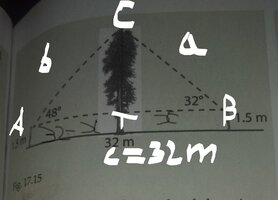That way you are thinking about the situation you are faced with and (especially in this case) are likely to realise the problem with the original diagram in the question!
Yes.
Please draw your own sketches in future (you can just use mine in this case).
Yes I will. You know I do. But for this one, I felt it wasn't necessary since a diagram has already being provided.
He could "check" it by calculating "h" using the much simpler approach outlined at Post #5 (q.v.)!

Did you not read all the way through that post (before you nicked my diagram?).

Are you deliberately trying to confuse the OP (even further)?
No, he does no such thing. I think I understand this method better than the sine rule you are suggesting: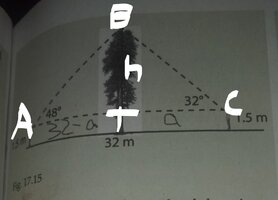from triangle ABT $tan(48)=\frac{h}{32-a}$from triangle BTC $tan(32)=\frac{h}{a}$$(32-a)tan(48)=h$$atan(32) = h$$a=\frac{32tan(48)-h}{tan(48)}$$a=\frac{h}{tan(32)}$$\frac{32tan(48)-h}{tan(48)}=\frac{h}{tan(32)}$$h=\frac{tan(32)\times 32tan(48)}{tan(48)+tan(32)}$$h=\frac{0.624869351\times1.110612515}{1.110612515+0.624869351} \\ = \frac{22.20760712}{1.735481867}$$h=12.79621962$Complete height of tree $=12.79621962+1.5 \\ \approx 14.3 m$To evaluate distance of the boy looking at the top of tree from the tree through an angle of elevation of 32 degree:
From triangle BTC, $a=\frac{h}{tan(32)}$$a=\frac{12.79621962}{0.624869351} \approx 20.5m$$\therefore$ the boy is standing at distance of 20.5m from the tree.
To evaluate distance of the boy looking at the top of tree from the tree through an angle of elevation of 48 degree:
$32-20.47823213 \approx 11.5 m$The last guy is standing at distance of 11.5 m from the tree.

#### khansaheb

##### Full Member
So could you please explain how $$\displaystyle \frac{32tan(48) \times tan(32)}{tan(32)+tan(48)}$$ gave rise to
Use pencil/paper .

Let
A = tan (48) ..... &....... B = tan(32).............Then.

32 * A * B/ ( A + B)...............divide numerator and denominator by (A * B) .......... I trust you know how to do that

= 32 * 1/[(1/A) + (1/B)] ......... I trust you can continue using the definition cot(x) = 1/tan(x)

Last edited:

#### The Highlander

##### Full Member
Your answers (for the height of the tree and the distance of each boy from it) now agree with mine (to the level of accuracy you have provided)....for this one, I felt it wasn't necessary since a diagram has already being provided.
It is always a good idea to draw your own sketch of a problem; it aids/reinforces your understanding of it.
(And the diagram "
provided" led to your mistakes in the first place!)
I think I understand this method better than the sine rule you are suggesting:
You are, of course, entitled to use whatever method you feel most comfortable with but you still appear to be missing my point about the use of the equals sign!
$h=\frac{tan(32)\times 32tan(48)}{tan(48)+tan(32)}$
may be true, but...
$h=\frac{0.624869351\times1.110612515}{1.110612515+0.624869351}$
is not true, because $tan(32)\ne 0.624869351$ even to nine decimal places!

Any numerical value
(other than a few, like, for example, Sin 30° = 0.5 or Cos 0° = 1, which are exact values) that a calculator (or a table) provides for the "value" of a trigonometric ratio is simply an approximation!

That is why you are (often) encouraged to work with surds in calculations involving trigonometric ratios, so that accuracy is preserved.

Last edited:

#### khansaheb

##### Full Member
Or more precisely, how is 32tan(48)×tan(32)=3232tan(48) \times tan(32)=3232tan(48)×tan(32)=32 and tan(32)+tan(48)=cot(32)+cot(48)tan(32)+tan(48)=cot(32) + cot(48)tan(32)+tan(48)=cot(32)+cot(48)
If you have gone through the steps of #18 you will see that statement above (derived by is incorrect.